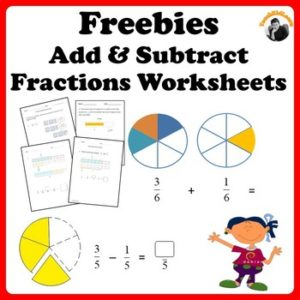# FREE MATH LESSON – “Fractions Worksheets Freebie Add, Subtract and Solve Word Problems”

by TeachKidLearnLearn and Practice Adding, Subtracting and Solving Fractions Word Problems

There are a total of 8 pages of worksheets in this Freebies.

Page 3-4: Subtracting Fractions
Page 6: Subtracting Mixed Numbers
Page 7-8: Solve Word Problems

Fraction diagrams are included to help students to add/subtract 2 fractions with same denominator as well as mixed numbers.

For adding/subtracting 2 fractions with different denominator, visual fraction models are provided to help students how to add/subtract 2 fractions with different denominator by applying equivalent fraction concept visually.

In solving word problems worksheets, 1 word problem comes with diagram while the other without diagram. You can challenge students/children to provide fraction diagram in the answer, if needed.

You can use these worksheets for students/children who are new to these fractions concept. Fraction visual diagrams will make it easy for them to understand.

Get the following individual resources or bundle pack for students/children to practice as they progress.

– add up to 3 fractions of same or different denominators.

(2)Subtracting Fractions
– add up to 3 fractions of same or different denominators.

– add and subtract mixed numbers with different denominators and regrouping.

(4)Solve Add and Subtract Fractions Word Problems
– Solve one and two steps word problems. Including fractions of same, different denominators and mixed numbers.

(5)Add and Subtract Fractions Worksheets Bundle Pack
– Comprises of all the above 4 fractions worksheets resources at a discount of 25%!

—————————————————————————————-

Based on Singapore mathematics approach.

—————————————————————————————-

Tips:

– Earn Credit for Future Purchases
Go to My Purchases page (you may need to login). Beside each purchase you’ll see a Provide Feedback Link. Simply click it and you will be taken to a page where you can give a quick rating and leave a short comment for the resource. Each time you give feedback, you receive feedback credits that you can use to lower the cost of your future purchases.
I value your feedback as it helps me determine which products are most useful and beneficial for you and your students.

– Be the First to Know Promo, Freebies and Product Launches

Join The Best of Teacher Entrepreneurs Marketing Cooperative at
http://www.thebestofteacherentrepreneursmarketingcooperative.com/2014/01/the-best-of-teacher-entrepreneurs.html
and get THOUSANDS OF PAGE VIEWS for your TpT products!

Go to http://www.pinterest.com/TheBestofTPT/ for even more free products!